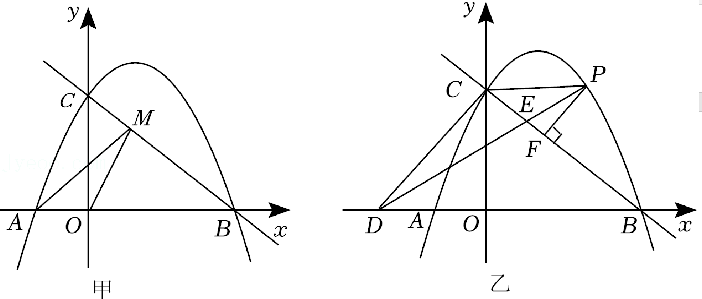(1) 求抛物线的解析式, 并直接写出点 $\mathrm{A}, \mathrm{C}$ 的坐标;
(2) 如图甲, 点 $\mathrm{M}$ 是直线 $\mathrm{BC}$ 上的一个动点, 连接 $\mathrm{AM}, \mathrm{OM}$, 是否存在点 $\mathrm{M}$ 使 $\mathrm{AM}+\mathrm{OM}$ 最小, 若存在, 请求出点 $\mathrm{M}$ 的坐标, 若 不存在, 请说明理由;
(3) 如图乙, 过点 $P$ 作 $P F \perp B C$, 垂足为 $F$, 过点 $C$ 作 $C D \perp B C$, 交 $\mathrm{x}$ 轴于点 $\mathrm{D}$, 连接 $\mathrm{DP}$ 交 $\mathrm{BC}$ 于点 $\mathrm{E}$, 连接 $C P$. 设 $\triangle \mathrm{PEF}$ 的面积为 $S_1, \triangle P E C$ 的面积为 $S_2$, 是否存在点 $P$, 使得 $\frac{S_1}{S_2}$ 最大, 若存在, 请求出点 $P$ 的坐标, 若不存在, 请说明理由.